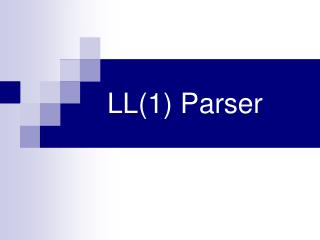DownloadDownload PresentationLL(1) Parser

# LL(1) Parser

Télécharger la présentation## LL(1) Parser

- - - - - - - - - - - - - - - - - - - - - - - - - - - E N D - - - - - - - - - - - - - - - - - - - - - - - - - - -
##### Presentation Transcript

1. LL(1) Parser

2. What does LL signify ? The first L means that the scanning takes place from Left to right. The second L means that the Left derivation is produced first. The prime requirements are : - • Stack • Parsing Table • Input buffer • Parsing program .

3. Input buffer contains the string to be parsed, followed by \$ ,a symbol used to indicate end of the input string. The stack indicates a sequence of grammar symbols with \$ on the bottom,indicating bottom of the stack. Initially, the stack contains the start symbol of the grammar on the top of \$. The parsing table is a 2-D array M[A,a] where A is a nonterminal, and a is a terminal or the symbol \$.

4. How to control the parser? • If X=a=\$ , parser halts, string accepted. • If X=a !=\$ , parser pops X, and advances the input pointer to point to next input symbol. • If X is a non-terminal, the program consults entry M[X,a] of the parsing table M. Replace the top of stack(X) with production rule corresponding to entry in table. If entry = ERROR, call error recovery routine.

5. Algo for Construction of predictive parsing table : • For each production Aa of grammar G, do steps 2 and 3 • For each terminal 'a' in FIRST(a) , add Aa in M[A,a]. • If e is in FIRST(a) , add Aa to M[A,b] for each terminal b in FOLLOW(A). If ë is in FIRST(a ) , and \$ is in FOLLOW(A), then add Aa to M[A,\$] • Make each undefined entry as “ERROR”, i.e. An error entry.

6. Example: Grammar ETE' E'+TE' | ë T FT' T'*FT' | ë F(E) | id ( ë stands for epsilon)

7. First and Follow

8. Building the table

9. Input=id+id*id

10. Thus, we can easily construct an LL parse with 1 lookahead. Since one look ahead is involved, we also call it an LL(1) parser. There are grammars which may requite LL(k) parsing. For e.g. look at next example…..

11. Grammar: SiEtSS’ | a S’Es | ë Eb Note that this is If then else statement

12. Parse Table Ambiguity

13. The grammar is ambiguous and it is evident by the fact that we have two entries corresponding to M[S’,e] containing S € and S’ eS. This ambiguity can be resolved if we choose S’eS i.e associating the else’s with the closest previous “then”.

14. Note that the ambiguity will be solved if we use LL(2) parser, i.e. always see for the two input symbols. How? When input is ‘e’ then it looks at next input. Depending on the next input we choose the appropriate rule.

15. LL(1) grammars have distinct properties. -No ambiguous grammar or left recursive grammar can be LL(1). A grammar is LL(1) if and only if whenever a production A C | D the following conditions hold: …contd

16. 1)For no terminal a both C and D derive strings beginning with a. Thus First(C) != First(D) 2)At most one of C or D can derive € 3) If C* € then D does not derive any string beginning with terminal Follow(A).

17. Prepared by Mukesh Kumar B 04CS1013,CSE, IIT Kharagpur. Visit: http://www.facweb.iitkgp.ernet.in/~niloy/COURSE/Autumn2006/Compiler/main.html#Lecture THE END